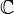# Cristalline Deligne cohomology

• ## General Introduction

This is notion used in this preprint of Vologodsky: Some applications of Goodwillie's theorem. It is a cohomology theory for schemes over, which agrees with usual Deligne cohomology when the scheme is smooth. The preprint studies infinitesimal deformations of varieties and questions of liftings of K-classes, using Hodge theory.

<]]>

<]]>

<]]>

<]]>

<]]>

<]]>

<]]>

<]]>

<]]>

<]]>

<]]>

<]]>

<]]>